# Forward-Backward Proximal Splitting¶

Important: Please read the installation page for details about how to install the toolboxes. $\newcommand{\dotp}{\langle #1, #2 \rangle}$ $\newcommand{\enscond}{\lbrace #1, #2 \rbrace}$ $\newcommand{\pd}{ \frac{ \partial #1}{\partial #2} }$ $\newcommand{\umin}{\underset{#1}{\min}\;}$ $\newcommand{\umax}{\underset{#1}{\max}\;}$ $\newcommand{\umin}{\underset{#1}{\min}\;}$ $\newcommand{\uargmin}{\underset{#1}{argmin}\;}$ $\newcommand{\norm}{\|#1\|}$ $\newcommand{\abs}{\left|#1\right|}$ $\newcommand{\choice}{ \left\{ \begin{array}{l} #1 \end{array} \right. }$ $\newcommand{\pa}{\left(#1\right)}$ $\newcommand{\diag}{{diag}\left( #1 \right)}$ $\newcommand{\qandq}{\quad\text{and}\quad}$ $\newcommand{\qwhereq}{\quad\text{where}\quad}$ $\newcommand{\qifq}{ \quad \text{if} \quad }$ $\newcommand{\qarrq}{ \quad \Longrightarrow \quad }$ $\newcommand{\ZZ}{\mathbb{Z}}$ $\newcommand{\CC}{\mathbb{C}}$ $\newcommand{\RR}{\mathbb{R}}$ $\newcommand{\EE}{\mathbb{E}}$ $\newcommand{\Zz}{\mathcal{Z}}$ $\newcommand{\Ww}{\mathcal{W}}$ $\newcommand{\Vv}{\mathcal{V}}$ $\newcommand{\Nn}{\mathcal{N}}$ $\newcommand{\NN}{\mathcal{N}}$ $\newcommand{\Hh}{\mathcal{H}}$ $\newcommand{\Bb}{\mathcal{B}}$ $\newcommand{\Ee}{\mathcal{E}}$ $\newcommand{\Cc}{\mathcal{C}}$ $\newcommand{\Gg}{\mathcal{G}}$ $\newcommand{\Ss}{\mathcal{S}}$ $\newcommand{\Pp}{\mathcal{P}}$ $\newcommand{\Ff}{\mathcal{F}}$ $\newcommand{\Xx}{\mathcal{X}}$ $\newcommand{\Mm}{\mathcal{M}}$ $\newcommand{\Ii}{\mathcal{I}}$ $\newcommand{\Dd}{\mathcal{D}}$ $\newcommand{\Ll}{\mathcal{L}}$ $\newcommand{\Tt}{\mathcal{T}}$ $\newcommand{\si}{\sigma}$ $\newcommand{\al}{\alpha}$ $\newcommand{\la}{\lambda}$ $\newcommand{\ga}{\gamma}$ $\newcommand{\Ga}{\Gamma}$ $\newcommand{\La}{\Lambda}$ $\newcommand{\si}{\sigma}$ $\newcommand{\Si}{\Sigma}$ $\newcommand{\be}{\beta}$ $\newcommand{\de}{\delta}$ $\newcommand{\De}{\Delta}$ $\newcommand{\phi}{\varphi}$ $\newcommand{\th}{\theta}$ $\newcommand{\om}{\omega}$ $\newcommand{\Om}{\Omega}$

This numerical tour presents the Forward-Backward (FB) algorithm to minimize the sum of a smooth and a simple function. It shows an application to sparse-spikes deconvolution.

In :
addpath('toolbox_signal')


## Bibliography¶

Excellent review papers on proximal splitting algorithms include:

Amir Beck and Marc Teboulle, Gradient-Based Algorithms with Applications to Signal Recovery Problems, in "Convex Optimization in Signal Processing and Communications". Editors: Yonina Eldar and Daniel Palomar. Cambridge university press.

P. L. Combettes and J.-C. Pesquet, Proximal splitting methods in signal processing, in: Fixed-Point Algorithms for Inverse Problems in Science and Engineering, (H. H. Bauschke, R. S. Burachik, P. L. Combettes, V. Elser, D. R. Luke, and H. Wolkowicz, Editors), pp. 185-212. Springer, New York, 2011.

## Forward-Backward Algorithm¶

We consider the problem of minimizing the sum of two functions $$E^\star \umin{x \in \RR^N} E(x) = f(x) + g(x).$$

We assume that $f$ is a $C^1$ function with $L$-Lipschitz gradient, which means that $$\forall (x,y) \in \RR^N \times \RR^N, \quad \norm{\nabla f(x) - \nabla f(y)} \leq L \norm{x-y}.$$

We also assume that $g$ is "simple", in the sense that one can compute in closed form the so-called proximal mapping, which is defined as $$\text{prox}_{\ga g}(x) = \uargmin{y \in \RR^N} \frac{1}{2}\norm{x-y}^2 + \ga g(y).$$ for any $\ga > 0$.

The FB algorithm reads, after initilizing $x^{(0)} \in \RR^N$, $$x^{(\ell+1)} = \text{prox}_{\ga g}\pa{ x^{(\ell)} - \ga \nabla f( x^{(\ell)} ) }.$$

If $0 < \ga < 2/L$, then this scheme converges to a minimizer of $f+g$.

## Sparse Regularization of Inverse Problems¶

We consider a linear inverse problem $$y = \Phi x_0 + w \in \RR^P$$ where $x_0 \in \RR^N$ is the (unknown) signal to recover, $w \in \RR^P$ is a noise vector, and $\Phi \in \RR^{P \times N}$ models the acquisition device.

To recover an approximation of the signal $x_0$, we use the Basis Pursuit denoising method, that use the $\ell^1$ norm as a sparsity enforcing penalty $$\umin{x \in \RR^N} \frac{1}{2} \norm{y-\Phi x}^2 + \la \norm{x}_1,$$ where the $\ell^1$ norm is defined as $$\norm{x}_1 = \sum_i \abs{x_i}.$$

The parameter $\la$ should be set in accordance to the noise level $\norm{w}$.

This minimization problem can be cast in the form of minimizing $f+g$ where $$f(x) = \frac{1}{2} \norm{y-\Phi x}^2 \qandq g(x) = \la \norm{x}_1.$$

$f$ is a smooth function, which satisfies $$\nabla f(x) = \Phi^* (\Phi x - y),$$ it has a $L$-Lipschitz gradient with $$L = \norm{ \Phi^* \Phi }.$$

The $\ell^1$-norm is "simple", because its proximal operator is a soft thresholding: $$\text{prox}_{\ga g}(x)_k = \max\pa{ 0, 1 - \frac{\la \ga}{\abs{x_k}} } x_k.$$

## Signal Deconvolution Problem on Synthetic Sparse Data¶

A simple linearized model of seismic acquisition consider a linear operator which is a filtering $$\Phi x = \phi \star x$$

Dimension of the problem.

In :
N = 1024;


We load a seismic filter $\phi$, which is a second derivative of a Gaussian.

Width of the filter.

In :
s = 5;


Second derivative of Gaussian.

In :
t = (-N/2:N/2-1)';
h = (1-t.^2/s^2).*exp( -(t.^2)/(2*s^2) );
h = h-mean(h);


Define the operator $\Phi$.

In :
h1 = fftshift(h); % Recenter the filter for fft use.
Phi = @(u)real(ifft(fft(h1).*fft(u)));


Display the filter and its Fourier transform.

Fourier transform (normalized)

In :
hf = real(fftshift(fft(h1))) / sqrt(N);


display

In :
q = 200;
clf;
subplot(2,1,1);
plot(-N/2+1:N/2, h);
axis([-q q min(h) max(h)]);
title('Filter, Spacial (zoom)');
subplot(2,1,2);
plot(-N/2+1:N/2, hf);
axis([-q q 0 max(hf)]);
title('Filter, Fourier (zoom)');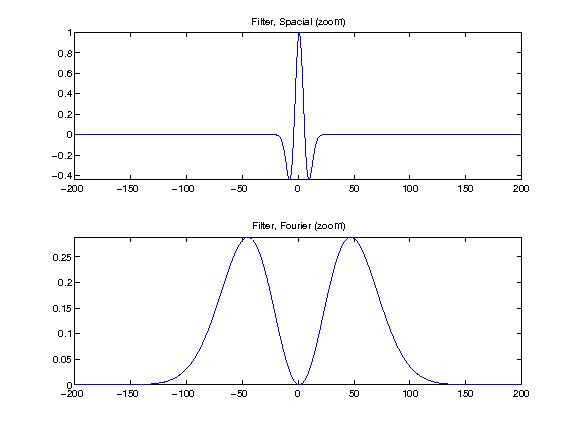We generate a synthetic sparse signal $x_0$, with only a small number of non zero coefficients.

Number of Diracs of the signal.

In :
s = round(N*.03);


Set the seed-number (for reproductibility).

In :
rand('state', 1);
randn('state', 1);


Location of the diracs.

In :
sel = randperm(N); sel = sel(1:s);


Signal $x_0$.

In :
x0 = zeros(N,1); x0(sel) = 1;
x0 = x0 .* sign(randn(N,1)) .* (1-.3*rand(N,1));


Noise level.

In :
sigma = .06;


Compute the measurements $y=\Phi x_0 + w$ where $w$ is a realization of a Gaussian white noise of variance $\si^2$.

In :
y = Phi(x0) + sigma*randn(N,1);


Display signals and measurements.

In :
clf; ms = 20;
subplot(2,1,1);
u = x0; u(x0==0) = NaN;
stem(u, 'b.', 'MarkerSize', ms); axis('tight');
title('Signal x_0');
subplot(2,1,2);
plot(y); axis('tight');
title('Measurements y');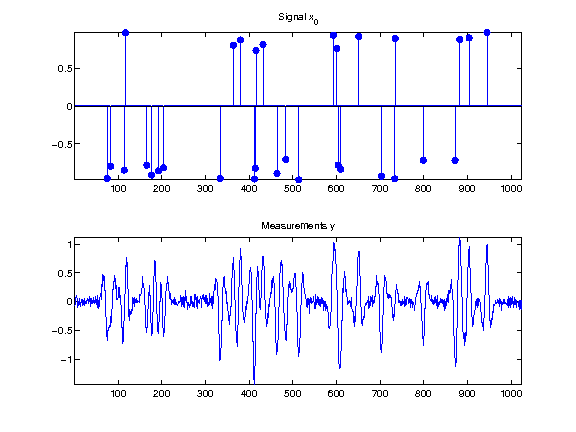## Sparse-Spikes Deconvolution¶

We now implement the FB algorithm for the sparse spikes problem.

Regularization strenght $\la$.

In :
lambda = 1;


Define the proximity operator of $\ga g$.

In :
proxg = @(x,gamma)perform_thresholding(x, lambda*gamma, 'soft');


Define the gradient operator of $f$

In :
gradf = @(x)Phi(Phi(x)-y);


Define the Lipschitz constant of $f$.

In :
L = max(abs(fft(h)))^2;


Gradient step size $\ga$, should be smaller than $2/L$.

In :
gamma = 1.95 / L;


Initialization $x^{(0)}$.

In :
x = y;


Perform one step of FB.

In :
x = proxg( x - gamma*gradf(x), gamma );


Exercise 1

Compute the solution of L1 deconvolution. Keep track of the degay of the energy $E=f+g$. isplay energy decay

In :
exo1()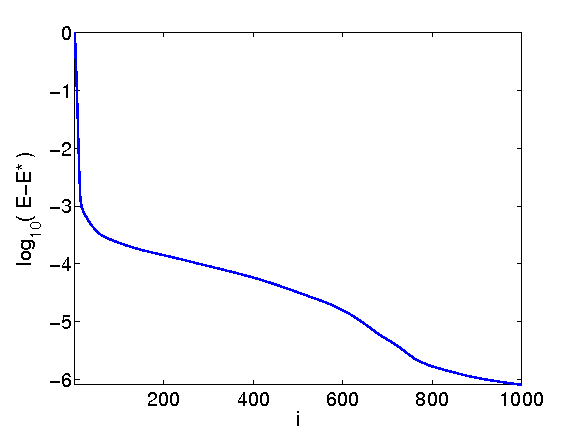In :
%% Insert your code here.


Display the result.

In :
clf;
subplot(2,1,1);
u = x0; u(x0==0) = NaN;
stem(u, 'b.', 'MarkerSize', ms); axis('tight');
title(['Signal x0']);
subplot(2,1,2);
u = x; u(x==0) = NaN;
stem(u, 'b.', 'MarkerSize', ms); axis('tight');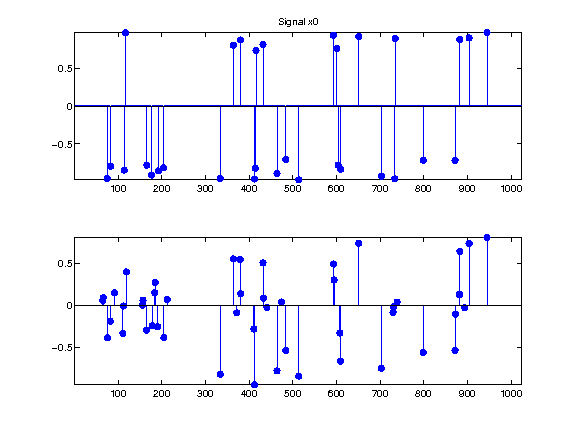## Over-relaxed Forward-Backward¶

It is possible to introduce a relaxation parameter $-1 < \mu < 1$ to average the current iterate of the FB with the previous one. In this case, one must set the descent paramter to $$\ga=\frac{1}{L}.$$

In :
gamma = 1/L;


The algorithm initializes $z^{(1)}=x^{(0)} \in \RR^N$, and then it computes $$x^{(\ell)} = \text{prox}_{\ga g}\pa{ z^{(\ell)} - \ga \nabla f( z^{(\ell)} ) }.$$ $$z^{(\ell+1)} = x^{(\ell)} + \mu \pa{ x^{(\ell)} - x^{(\ell-1)} }$$

The regime $-1<\mu <0$ corresponds to under-relaxation. Setting $\mu=0$, one recovers the unrelaxed Forward-Backward. Setting $0 < \mu < 1$ creates over-relaxation.

Note that convergence of the iterates $x^{(\ell)}$ is only guaranteed for $-1 < \mu < 1/2$, and that one can only prove convergence of $E(x^{(\ell)})$ toward $E^\star$ in case $\mu \in [1/2,1[$.

For a in-depth analysis of this scheme, see the book:

H.H. Bauschke and P.L. Combettes, Convex Analysis and Monotone Operator Theory in Hilbert Spaces Springer-Verlag (2011)

Exercise 2

Impement the relaxed FB algorithm and display its convergence rate for several values of $\mu$. isplay

In :
exo2()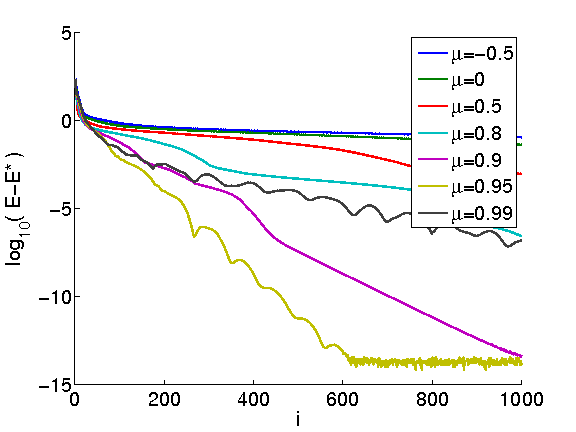In :
%% Insert your code here.


## FISTA Accelerated Algorithm¶

It is possible to use an adaptive relaxation parameter $\mu = \mu^{(\ell)}$ that changes from iteration to iteration.

This strategy is used in the Fast Iterative Soft Thresholding (FISTA) algorithm introduced in:

Amir Beck and Marc Teboulle, A Fast Iterative Shrinkage-Thresholding Algorithm for Linear Inverse Problems, SIAM Journal on Imaging Sciences 2 (2009), no. 1, 183--202.

In this algorithm, the relaxation parameters $\mu^{(\ell)}$ are automatically set and tend to the limit value $$\mu^{(\ell)} \overset{\ell \rightarrow +\infty}{\longrightarrow} 1 .$$

The step size should be set to: $$\ga = \frac{1}{L}.$$

In :
gamma = 1/L;


The algorithm initializes $z^{(1)}=x^{(0)} \in \RR^N$, and $t^{(1)}=1$ and then it computes $$x^{(\ell)} = \text{prox}_{\ga g}\pa{ z^{(\ell)} - \ga \nabla f( z^{(\ell)} ) }.$$ $$t^{(\ell+1)} = \frac{ 1 + \sqrt{1+4(t^{(\ell)})^2} }{2} \qandq \mu^{(\ell+1)} = \frac{t^{(\ell)}-1}{ t^{(\ell+1)} }$$ $$z^{(\ell+1)} = x^{(\ell+1)} + \mu^{(\ell+1)} \pa{ x^{(\ell)} - x^{(\ell-1)} }$$

For this scheme, one cannot prove convergence of the iterates, but one can prove that it reaches an optimal convergence rate of the iterates, namely $$E(f^{(\ell)}) - E^\star = O(1/\ell^2)$$ while the convergence rate for the usual FB is only $O(1/\ell)$.

Exercise 3

Compute the solution of L1 deconvolution using FISTA. Keep track of the degay of the energy $E = f+g$. isplay energy decay

In :
exo3()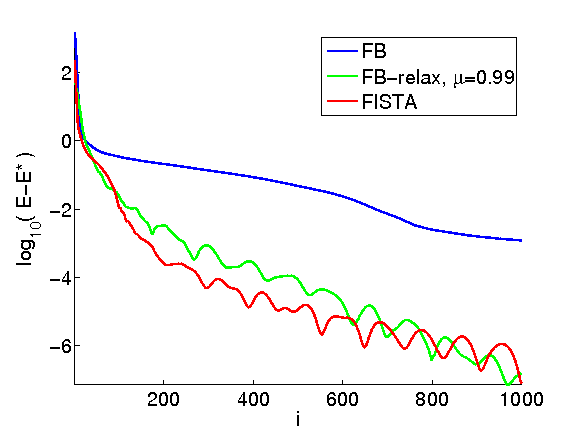In :
%% Insert your code here.